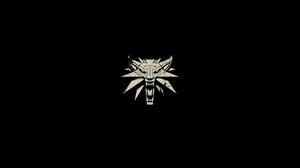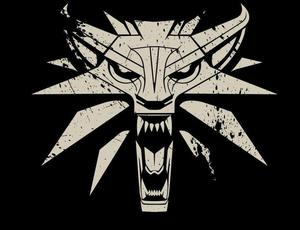# How to Crop an Image using the Numpy Module?

• Last Updated : 12 Nov, 2020

In this article, we are going to learn about the most naive and efficient approach to crop an image without using any additional module.

The numpy module is a Python library used for working with arrays, and large data sets. Python does not have any native support for arrays, as opposed to other high-level languages such as C, C++, Java, etc. which provides an implementation for arrays natively. Python rather has Linked lists, which does solve the problem for static allocation (mostly) and allows for storage of heterogeneous data, but does not allow for contiguous storage of data. Numpy makes up for this drawback by introducing arrays in the language which are a homogeneous data structure and are stored in contiguous memory locations.

Since the data inside an image (excluding the header info) is homogeneous, and are generally accessed sequentially or by direct access (via adding offsets), using arrays for storing image pixel data is allows for faster operations on the image. In this article, we will take a look at cropping an image using Numpy arrays (containing pixel information).

There are various methods in many modules to crop an image, the most naive and efficient approach to crop an image is to use indexing of numpy arrays.

## Using Indexing for Cropping Images

Since Numpy doesn’t support the image cropping method natively (since it not being an image processing library), we can use the indexing methods to fulfill our purpose. For Demonstration, we would be using the following image:-The following image is has 4K (3840×2160) dimensions.

We would be cropping the above image such that the logo in the middle occupies a large part of the image.

Since cropping image is *generally a manual thing to do, we would have to get the coordinates to the Region of Interest beforehand. 4 coordinates (or a pair of size 2 tuples) are required for cropping. The first set of coordinates specify the Top left corner of the ROI (or Bbox) and the next two denote the bottom right corner coordinates of the ROI. For our case the coordinates for the ROI would be (1413, 653) and (2361, 1385) (assuming row-major indexing is used).  For displaying and reading the image, we would be taking the help of Pillow library, which is an image processing library in python.

Below is the program to crop a given image:

## Python3

 `# Import required modules``from` `PIL ``import` `Image``import` `numpy as np`` ` `# Load image``image ``=` `Image.``open``(``'W3.jpg'``)`` ` `# Convert image to array``image_arr ``=` `numpy.array(image)`` ` `# Crop image``image_arr ``=` `image_arr[``700``:``1400``, ``1450``:``2361``]`` ` `# Convert array to image``image ``=` `Image.fromarray(image_arr)`` ` `# Display image``image.show()`

Output:Explanation:

• Firstly we imported the Image module of the PIL (or pillow) library. Then we imported the Numpy library under the alias np (common convention). After which we created an Image object of our desired image (W3.jpg), and stored the object in the variable image. So, the image variable is of type PIL.JpegImagePlugin.JpegImageFile.
• To create Numpy array out of this object, we passed it through the np.array() method, which extracted all the Pixel data from the image and stored it in the variable image_arr. This resulted us in having a Numpy array of shape (2160, 3840, 3)
• Then we sliced the array from each dimension. In the statement image_arr[700:1400, 1450:2361] the 700 is denoting the starting row, and 1400 is denoting the ending row. Where, 1450 represents starting column, and 2316 represents the ending column. All these values depict the pixel at the location, therefore the crop with the top left coordinates of (1450, 700) and bottom left coordinates of (2361, 1400)
• Finally, we converted the Numpy array back to an image using Image.fromarray(). In the end we displayed the image using the show() function.
My Personal Notes arrow_drop_up